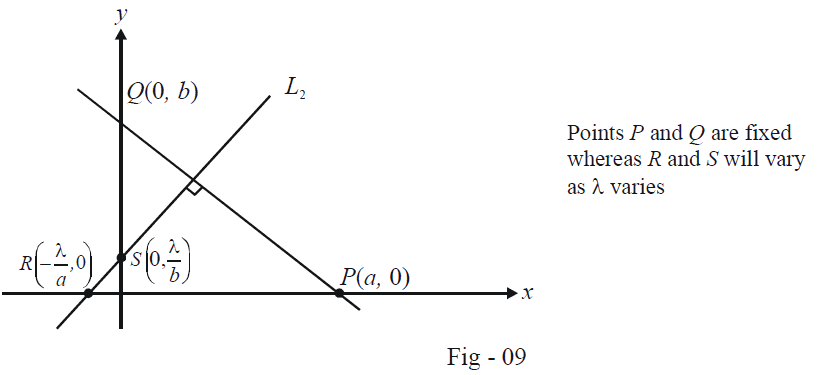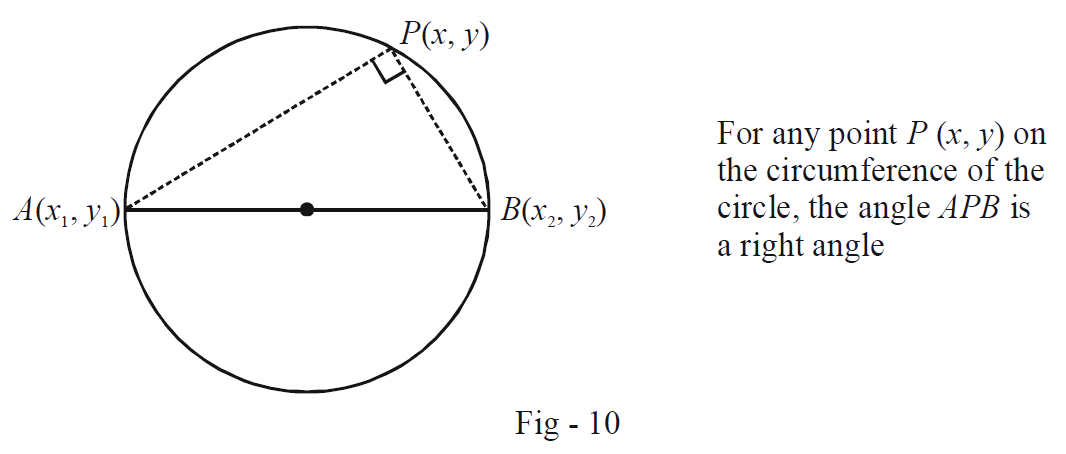# Jee Examples On Equations Of Circles Set-2

Go back to  'Circles'

Example -10

A fixed line $$L_1$$  intersects the co-ordinate axes at $$P(a, 0)$$ and $$Q (0, b)$$. A variable line $$L_2$$, perpendicular to $$L_1$$, intersects the axes at $$R$$ and $$S$$. Show that the locus of the points of intersection of $$PS$$ and $$QR$$ is a circle.

Solution: The equation of $$L_1$$, using intercept form, can be written as

\begin{align} &\frac{x}{a} + \frac{y}{b} = 1\\ \Rightarrow \qquad & bx + ay = ab \end{align}

Since $$L_2$$ is perpendicular to $$L_1$$, its equation can be written as

${L_2} \equiv ax - by + \lambda = 0$

where \begin{align}\lambda\end{align} is a real parameter.

Using the equation of $$L_2$$, we can determine $$R$$ and $$S$$ to be \begin{align}\left( { - \frac{\lambda }{a},0} \right)\;{\rm{and}}\;\left( {0,\frac{\lambda }{b}} \right)\end{align} respectively.We now write the equations to $$PS$$ and $$QR$$ using the two-point form:

\begin{align} &PS:\frac{y}{{x - a}} = \frac{{ - \lambda }}{{ab}} \qquad \quad \Rightarrow \qquad \quad \lambda x + aby = a\lambda \qquad \qquad \qquad ...(1)\\ &QR:\frac{{y - b}}{x} = \frac{{ab}}{\lambda } \qquad \quad \;\, \Rightarrow \qquad \quad - abx + \lambda y = b\lambda \qquad \qquad \quad \; ...(2) \end{align}

The relation that the intersection point of $$PS$$ and $$QR$$, will satisfy can be evaluated by eliminating \begin{align}\lambda \end{align} from (1) and (2). We thus obtain

\begin{align} & \lambda = \frac{{aby}}{{a - x}} = \frac{{abx}}{{y - b}}\\ \Rightarrow \qquad & ab{y^2} - a{b^2}y = {a^2}bx - ab{x^2}\\ \Rightarrow \qquad & {x^2} + {y^2} - ax - by = 0 \end{align}

This represents a circle centered at \begin{align}\left( {\frac{a}{2},\frac{b}{2}} \right)\end{align} and passing through the origin.

Example -11

Let \begin{align}\left( {{m_i},\frac{1}{{{m_i}}}} \right),\,i = 1,\,\,2,\,\,3,\,\,4\end{align} be four distinct points lying on a circle. Prove that \begin{align}{m_1}{m_2}{m_3}{m_4} = 1\end{align}

Solution: We first assume an equation for this circle $$C$$, in its general form:

$C:{x^2} + {y^2} + 2gx + 2fy + c = 0$

Since \begin{align}\left( {{m_i},\frac{1}{{{m_i}}}} \right)\end{align} satisfies the equation of $$C$$ for $$i = 1, 2, 3, 4$$ we have

\begin{align} & m_i^2 + \frac{1}{{m_i^2}} + 2g{m_i} + \frac{{2f}}{{{m_i}}} + c = 0 \qquad \qquad i = 1,\,2,\,3,\,4\\ \Rightarrow \qquad & m_i^4 + 2gm_i^3 + cm_i^2 + 2f{m_i} + 1 = 0 \qquad \quad \; i = 1,\,\,2,\,\,3,\,\,4 \end{align}

This last equation tells us that  $${{m}_{i}}s$$  are the roots of the following equation in $$m$$:

\begin{align}{m^4} + 2g{m^3} + c{m^2} + 2fm + 1 = 0: & {\rm{Roots}}\;{\rm{of}}\;{\rm{this}}\;{\rm{equation}}\;{\rm{are}}\;m,\;i = 1,\;2,\;3,\;4\end{align}

The product of the roots, which is \begin{align}{m_1}{m_2}{m_3}{m_4}\end{align}, can easily be seen to be $$1$$ from this equation.

Example -12

Find the equation of the circle $$C$$ which has two fixed points \begin{align}A\left( {{x_1},{y_1}} \right)\,\,{\rm{and}}\,B\left( {{x_2},{y_2}} \right)\,\end{align}  as the end-points of its diameter.

Solution: To evaluate the required equation, we can use a well known result from plane geometry: the angle in a semicircle is a right angle.Thus, we can use this fact:

\begin{align} (Slope\;of\;AP) \times &(Slope\;of\;PB) = - 1\\ \Rightarrow \qquad & \frac{{y - {y_1}}}{{x - {x_1}}} \times \frac{{y - {y_2}}}{{x - {x_2}}} = - 1\\ \Rightarrow \qquad & \left( {x - {x_1}} \right)\left( {x - {x_2}} \right) + \left( {y - {y_1}} \right)\left( {y - {y_2}} \right) = 0 & & ...(1) \end{align}

This is the required equation that will represent $$C$$. Note that we could equivalently have used the Pythagoras theorem in \begin{align}\Delta APB\end{align} to evaluate the equation of $$C$$:

\begin{align} & A{P^2} + P{B^2} = A{B^2}\\ \Rightarrow \qquad & {\left( {x - {x_1}} \right)^2} + {\left( {y - {y_1}} \right)^2} + {\left( {x - {x_2}} \right)^2} + {\left( {y - {y_2}} \right)^2} = {\left( {{x_1} - {x_2}} \right)^2} + {\left( {{y_1} - {y_2}} \right)^2}\\ \Rightarrow \qquad & 2{x^2} + 2{y^2} - 2x\left( {{x_1} + {x_2}} \right) - 2y\left( {{y_1} + {y_2}} \right) = - 2{x_1}{x_2} - 2{y_1}{y_2}\\ \Rightarrow \qquad & \left( {x - {x_1}} \right)\left( {x - {x_2}} \right) + \left( {y - {y_1}} \right)\left( {y - {y_2}} \right) = 0 \end{align}

which is the same as what we obtained in (1).